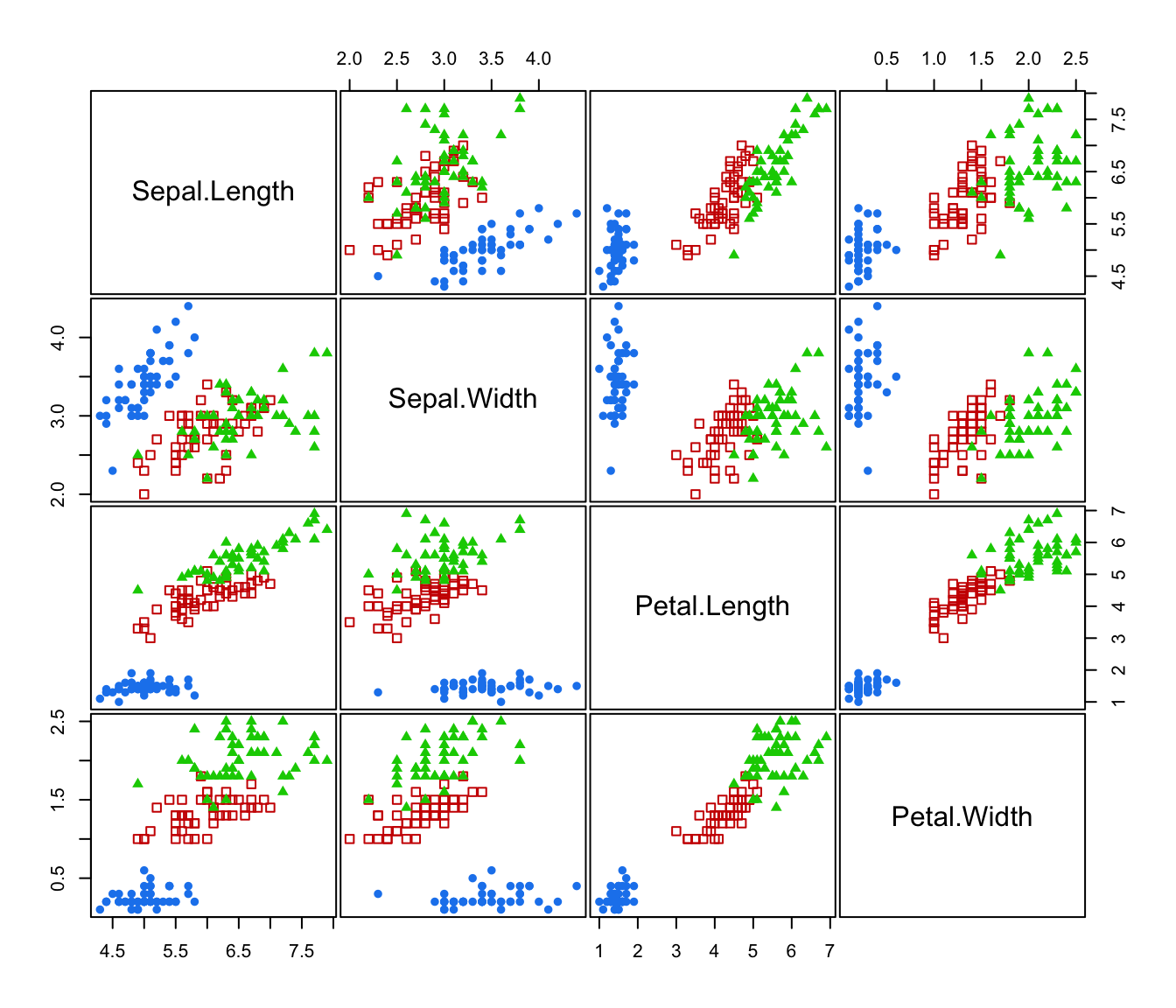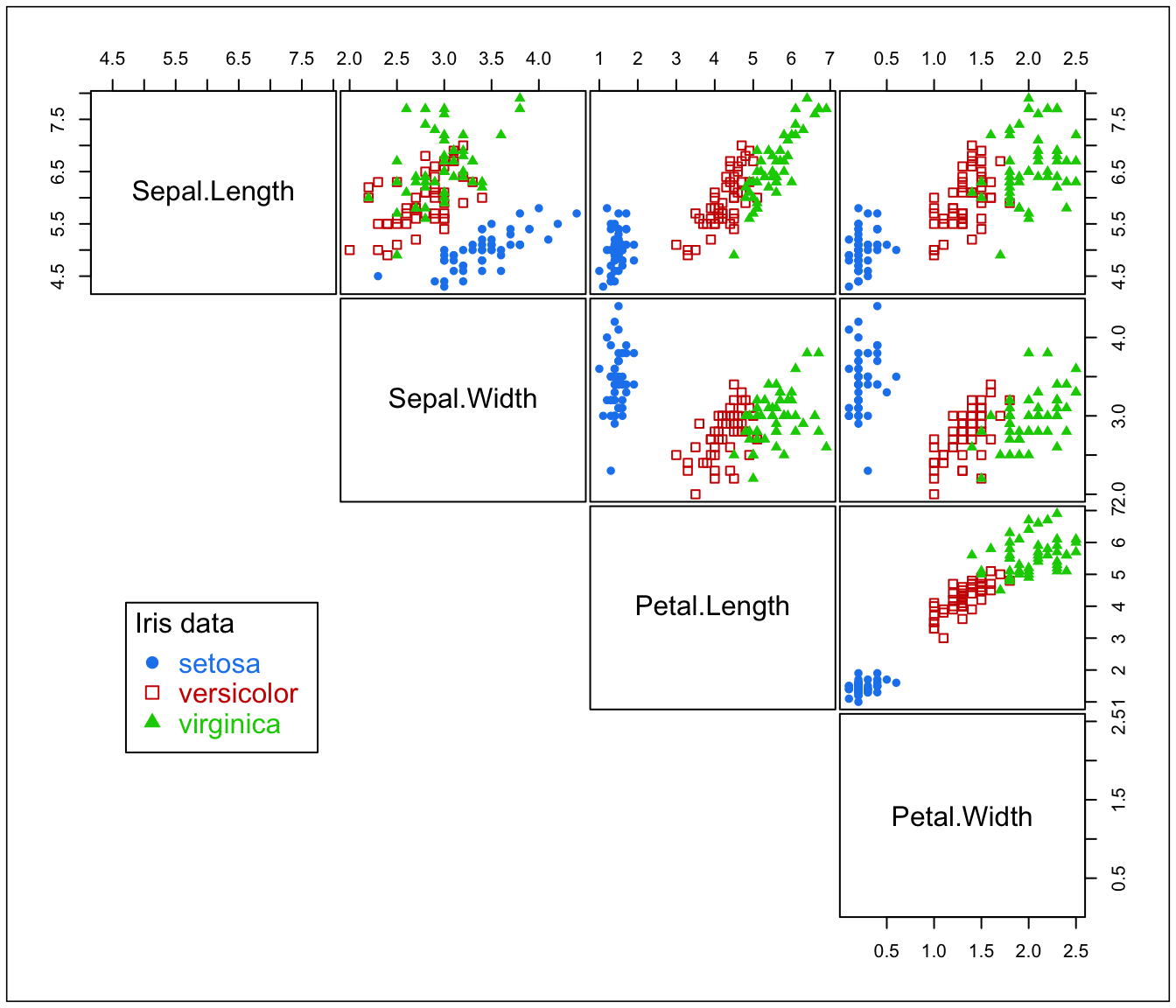Creates a scatter plot for each pair of variables in given data. Observations in different classes are represented by different colors and symbols.

## Usage

clPairs(data, classification,
symbols = NULL, colors = NULL, cex = NULL,
labels = dimnames(data)[], cex.labels = 1.5,
gap = 0.2, grid = FALSE, ...)

clPairsLegend(x, y, class, col, pch, cex, box = TRUE, ...)

## Arguments

data

A numeric vector, matrix, or data frame of observations. Categorical variables are not allowed. If a matrix or data frame, rows correspond to observations and columns correspond to variables.

classification

A numeric or character vector representing a classification of observations (rows) of data.

symbols

Either an integer or character vector assigning a plotting symbol to each unique class in classification. Elements in symbols correspond to classes in order of appearance in the sequence of observations (the order used by the function unique). The default is given by mclust.options("classPlotSymbols").

colors

Either an integer or character vector assigning a color to each unique class in classification. Elements in colors correspond to classes in order of appearance in the sequence of observations (the order used by the function unique). The default is given by mclust.options("classPlotColors").

cex

A vector of numerical values specifying the size of the plotting symbol for each unique class in classification. Values in cex correspond to classes in order of appearance in the sequence of observations (the order used by the function unique). By default cex = 1 for all classes is used.

labels

A vector of character strings for labelling the variables. The default is to use the column dimension names of data.

cex.labels

A numerical value specifying the size of the text labels.

gap

An argument specifying the distance between subplots (see pairs).

grid

A logical specifying if grid lines should be added to panels (see grid).

x,y

The x and y co-ordinates with respect to a graphic device having plotting region coordinates par("usr" = c(0,1,0,1)).

class

The class labels.

box

A logical, if TRUE then a box is drawn around the current plot figure.

col, pch

The colors and plotting symbols appearing in the legend.

...

For a clPairs call may be additional arguments to be passed to pairs. For a clPairsLegend call may be additional arguments to be passed to legend.

## Details

The function clPairs() draws scatter plots on the current graphics device for each combination of variables in data. Observations of different classifications are labeled with different symbols.

The function clPairsLegend() can be used to add a legend. See examples below.

## Value

The function clPairs() invisibly returns a list with the following components:

class

A character vector of class labels.

col

A vector of colors used for each class.

pch

A vector of plotting symbols used for each class.

pairs, coordProj, mclust.options
clPairs(iris[,1:4], cl = iris$Species)clp <- clPairs(iris[,1:4], cl = iris$Species, lower.panel = NULL)
clPairsLegend(0.1, 0.4, class = clp$class, col = clp$col, pch = clp\$pch,